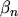## 6.2.1 Six Moments Transport Model

To obtain information about the distribution function additional to the average energy several authors proposed usage of higher order moment equations, see for example  . These equations were based on a distribution function expanded around a Maxwellian distribution. SONODA  added two equations for the fourth and fifths moment of BOLTZMANN's transport equation to a standard energy transport model, taken from . A more consistent approach has been presented in Chapter 2 where a transport model using six moments of BOLTZMANN's transport equation has been derived, with the kurtosisas an additional state variable [39, G5].

Although this approach appears promising from a physical point of view, it has not been used to tackle the SOI problem as the additional balance equation significantly increases numerical complexity and computation times. Convergence behavior also worsens considerably. Instead, the framework of energy transport equations was adopted and corrections accounting for distribution function effects were introduced.

M. Gritsch: Numerical Modeling of Silicon-on-Insulator MOSFETs PDF